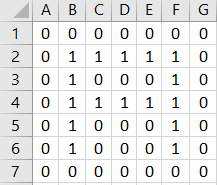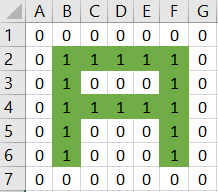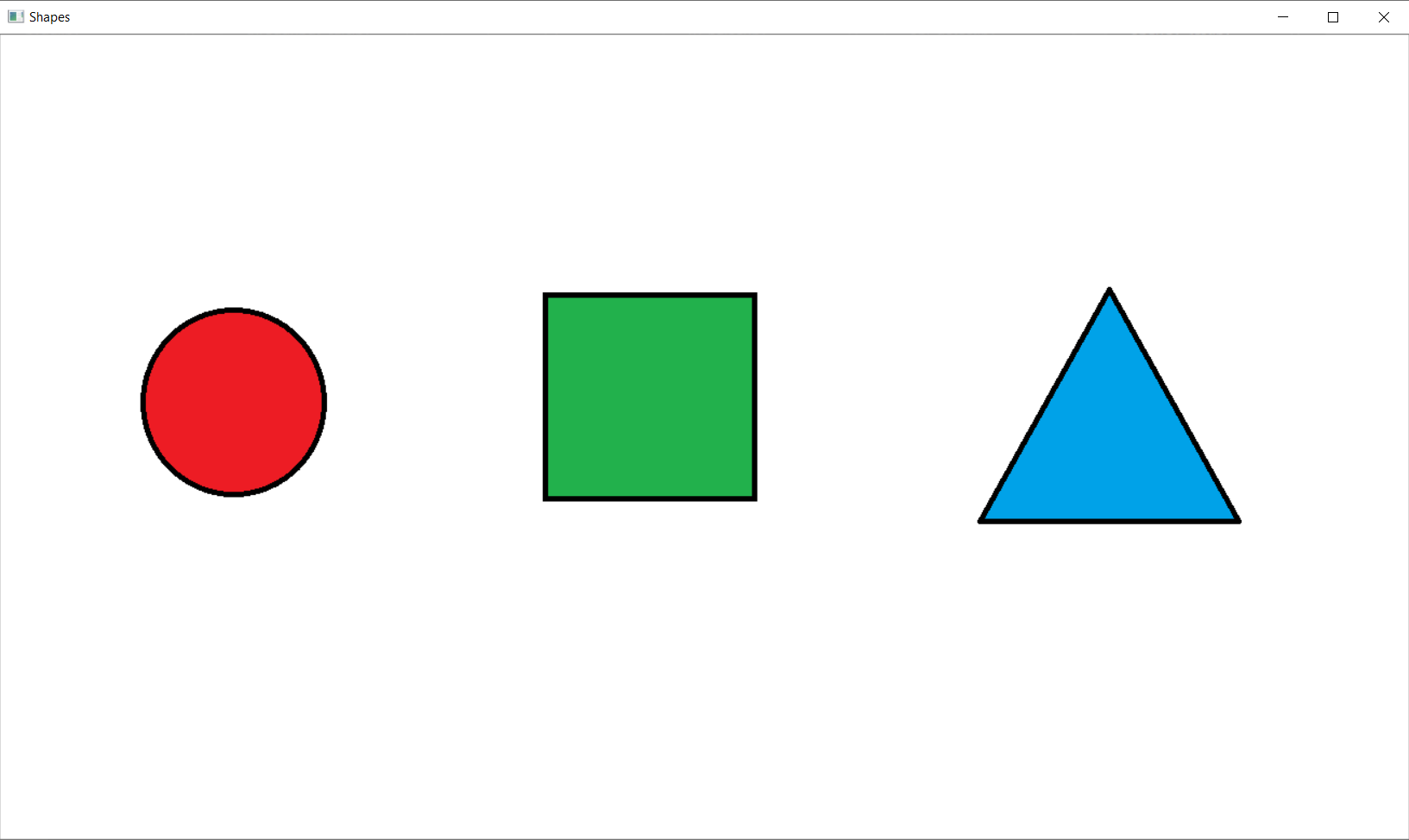OpenCV is a project that was started back in 1999 by Intel. The primary purpose? to achieve computer vision. In this article we will be taking a look at OpenCV-python, the python API for the OpenCV project.

This python library allows us to detect objects and shapes in both photos and images, as well as in a live camera feed. Before we dive into the workings of the OpenCV library, let us understand what an image is in the world of computers.

An image in computer vision is basically a binary matrix. A matrix that contains a series of meaningfully placed 1s and 0s. In the following image, see if you can discern the shape of the English alphabet 'A' by connecting the series of 1s in the matrix.Now that we know how outlines in an image are represented, lets take a look at how color is represented. Lets take a look at the RGB model which contains three primary colors that form the color space. This model contains 3 colors namely Red, Green and Blue, and hence the name RGB.

Each color is made up of 8 bits, that can have an integer value range from 0 to 255 each. Hence a total of 256 x 256 x 256 = 16777216 colors are possible using varying combinations of these colors.As can be seen above, any image can be represented in the form of an RBG matrix.

Now that we have a basic understanding of what an image is lets move on to exploring some of the basic functionalities that OpenCV provides us with. Before we begin, install the library using pip -

pip3 install opencv-python

As always, we'll explore the library as a series of tasks. Let us take the following sample image of a simple shapes to be detected.

For this process we will be using two main functions -

cv2.imshow() - To display the image

We'll also add a waitkey() function at the end so that the output of our code doesn't disappear as soon as the function execution ends. The value "0" indicates an infinite wait timer until the time a keyboard input is pressed.

```import cv2

cv2.imshow("Apple",img)

cv2.waitKey(0)```

The output for which would be -Task 2 - Converting the image to a grey-scale

To convert the image to a grey-scale we use a function called cv2.cvtColor(). Converting the image to gray-scale means converting a colored image into shades of grey. This is a concept that is useful for thresholding, a concept that we will see in subsequent sections in the tutorial.

```import cv2

cv2.imshow("Shapes",img)

#convert image to greyscale
imgGray = cv2.cvtColor(img,cv2.COLOR_BGR2GRAY)
cv2.imshow("Grey Image",imgGray)

cv2.waitKey(0) ```

The converted grey-scale would be as follows,

Task 3 - Using Thresholding and Contours to detect all the outlines

Threshold is a method of segmenting the image image based on a specific value or limit. A threshold value is basically a value that demarcates the segments that have been formed.

Contours are defined as the lines formed by connecting the pixels of same intensity or color along the boundaries of an image.

Now, in order to find all the contours present in an image, we will make use of 2 main OpenCV functions -

cv2.threshold() - To set the threshold value to segment the image on
cv2.findContours() - To find all the contours present in the image

The cv2.threshold() function takes the following arguments -

+ imgGray : The gray-scale image to be fed as input
+ 127 : Threshold value
+ 255 : The maximum value of the pixel range
+ 0 : setting the thresholding technique

The cv2.findContours() function takes the following arguments -

+ thresh : The returned image with the thresholding applied
+ cv2.RETR_TREE : The contour retrieval mode
+ cv2.CHAIN_APPROX_NONE : The contour approximation method

NOTE - Be sure to run the thresholding and findContours command on the grey-scale image.

```import cv2
imgGray = cv2.cvtColor(img,cv2.COLOR_BGR2GRAY)cv2.imshow("Gray Image",imgGray)
#Find all the contours in an image
ret,thresh = cv2.threshold(imgGray,127,255,0)
contours,heirarchy = cv2.findContours(thresh,cv2.RETR_TREE,cv2.CHAIN_APPROX_NONE)
print("Number of contours: ", str(len(contours)))
print(contours)
cv2.waitKey(0)
```

The output with the total number of contours and the numpy array of the boundry points would be as follows,

Task 4 - Detecting the shapes and drawing an outline around them

In this task we will make use of a function named drawContours() to draw a yellow outline outside all the shapes.

cv2.drawContours() - To draw the contours around all shapes in the image

Arguments -
+ img : The original image to draw the contour on
+ contours : The contours array we got from the previous task
+ -1 : The contour no. to be drawn. A value of -1 indicates that all the contours will be drawn
+ (0,255,255) : The color of the contour being drawn using the RGB model, which in this case is yellow.

+ 2 : The thickness of the contour being drawn

```import cv2

cv2.imshow("Shapes",img)

imgGray = cv2.cvtColor(img,cv2.COLOR_BGR2GRAY)
cv2.imshow("Grey Image",imgGray)

ret,thresh = cv2.threshold(imgGray,127,255,0)
contours,heirarchy = cv2.findContours(thresh,cv2.RETR_TREE,cv2.CHAIN_APPROX_NONE)
print("Number of contours: ", str(len(contours)))
print(contours)

#drawing contours around all the shapes

cv2.drawContours(img,contours,-1,(0,255,255),2)

cv2.imshow("Shapes",img)

cv2.waitKey(0)```

Which would give the following output -

Now that we have this output in hand, it will be a relatively easy process to detect each of the shapes.

We will use the no. of edges in each contour as a method for differentiating between the 3 shapes given above. We'll use 2 main functions to determine each of the shapes -

cv2.arcLength() - To find the perimeter of the contours
cv2.approxPolyDP() - To approximate a poligon's contours based on the specified parameters

The cv2.arcLength() function takes the following arguments -

+ c : Each contour in the list of recieved contours
+ True : To check if the contour is a closed figure or not

The cv2.approxPolyDP() function takes the following arguments -

+ c : Each contour in the list of recieved contours
+ 0005 * peri : A value called as epsilon specifying the approximation accuracy which is the maximum distance between the original contour and its approximation.
+True : To check if the contour is a closed figure or not

```import cv2

imgGray = cv2.cvtColor(img,cv2.COLOR_BGR2GRAY)

ret,thresh = cv2.threshold(imgGray,127,255,0)
contours,heirarchy = cv2.findContours(thresh,cv2.RETR_TREE,cv2.CHAIN_APPROX_NONE)
print("Number of contours: ", str(len(contours)))
print(contours)

for c in contours:
peri = cv2.arcLength(c, True)
approx = cv2.approxPolyDP(c, 0.005 * peri, True)

# To detect a triangle
if len(approx) == 3:
scrnCnt = approx
cv2.drawContours(img, [scrnCnt], -1, (0, 255, 0), 3)

# To detect a square
elif len(approx) == 4:
scrnCnt1 = approx
cv2.drawContours(img, [scrnCnt1], -1, (0, 0, 255), 3)

# To detect a circle
elif len(approx) > 5:
scrnCnt2 = approx
cv2.drawContours(img, [scrnCnt2], -1, (255, 0, 0), 3)

cv2.imshow("Shapes",img)
cv2.waitKey(0)```

Which will detect the following shapes for us -

We now have successfully detected all the 3 shapes in the images and drawn borders of different colors along their edges so as to identify them correctly.

Task 5 - Labelling the images detected

Now that we have successfully identified all the shapes in the image, it would be a good idea to label each detected shape with an appropriate name. For this purpose we would be using a function called as putText().

The cv2.putText() function takes in the following arguments -

+ img : The image to write the labels on
+ "Square" : The text to be written as a label
+ pos : The bottom right corner of the text being displayed called as org.
+ 3 : Font scale
+ cv2.FONT_HERSHEY_PLAIN : Font family
+ (0,0,0) : Font color

```import cv2

imgGray = cv2.cvtColor(img,cv2.COLOR_BGR2GRAY)

ret,thresh = cv2.threshold(imgGray,127,255,0)
contours,heirarchy = cv2.findContours(thresh,cv2.RETR_TREE,cv2.CHAIN_APPROX_NONE)

for c in contours:
peri = cv2.arcLength(c, True)
approx = cv2.approxPolyDP(c, 0.005 * peri, True)
pos = tuple(map(tuple,c))
print(pos)
print(type(pos))

# To detect a triangle
if len(approx) == 3:
scrnCnt = approx
cv2.drawContours(img, [scrnCnt], -1, (0, 255, 0), 3)
pos = tuple(map(tuple,c))
cv2.putText(img,"Triangle",pos,3,cv2.FONT_HERSHEY_PLAIN,(0,0,0))

# To detect a square
elif len(approx) == 4:
scrnCnt1 = approx
cv2.drawContours(img, [scrnCnt1], -1, (0, 0, 255), 3)
pos = tuple(map(tuple,c))
cv2.putText(img,"Square",pos,3,cv2.FONT_HERSHEY_PLAIN,(0,0,0))

# To detect a circle
elif len(approx) > 5:
scrnCnt2 = approx
cv2.drawContours(img, [scrnCnt2], -1, (255, 0, 0), 3)
pos = tuple(map(tuple,c))
cv2.putText(img,"Circle",pos,3,cv2.FONT_HERSHEY_PLAIN,(0,0,0))

cv2.imshow("Shapes",img)
cv2.waitKey(0)

```